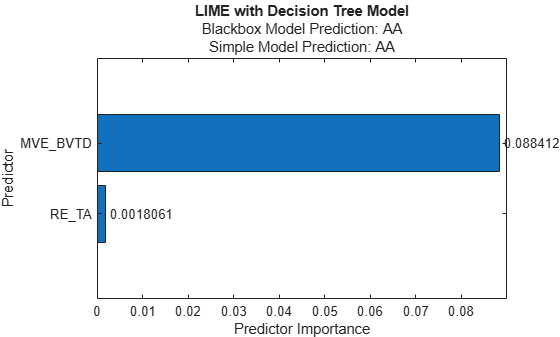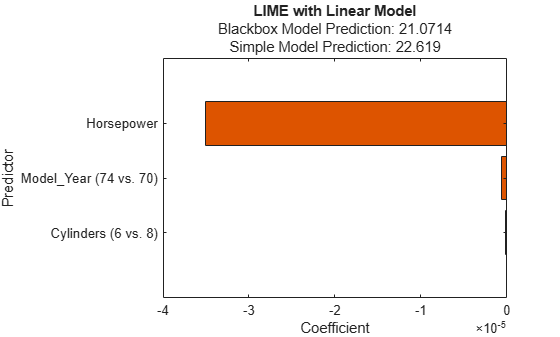# plot

Plot results of local interpretable model-agnostic explanations (LIME)

Since R2020b

## Syntax

``f = plot(results)``

## Description

example

````f = plot(results)` visualizes the LIME results in the `lime` object `results`. The function returns the `Figure` object `f`. Use `f` to query or modify Figure Properties of the figure after it is created. The figure contains a horizontal bar graph that shows the coefficient values of a linear simple model or predictor importance values of a decision tree simple model, depending on the simple model in `results` (`SimpleModel` property of `results`).The figure displays two predictions for the query point computed using the machine learning model and the simple model, respectively. These values correspond to the `BlackboxFitted` property and the `SimpleModelFitted` property of `results`. ```

## Examples

collapse all

Train a classification model and create a `lime` object that uses a decision tree simple model. When you create a `lime` object, specify a query point and the number of important predictors so that the software generates samples of a synthetic data set and fits a simple model for the query point with important predictors. Then display the estimated predictor importance in the simple model by using the object function `plot`.

Load the `CreditRating_Historical` data set. The data set contains customer IDs and their financial ratios, industry labels, and credit ratings.

`tbl = readtable('CreditRating_Historical.dat');`

Display the first three rows of the table.

`head(tbl,3)`
``` ID WC_TA RE_TA EBIT_TA MVE_BVTD S_TA Industry Rating _____ _____ _____ _______ ________ _____ ________ ______ 62394 0.013 0.104 0.036 0.447 0.142 3 {'BB'} 48608 0.232 0.335 0.062 1.969 0.281 8 {'A' } 42444 0.311 0.367 0.074 1.935 0.366 1 {'A' } ```

Create a table of predictor variables by removing the columns of customer IDs and ratings from `tbl`.

`tblX = removevars(tbl,["ID","Rating"]);`

Train a blackbox model of credit ratings by using the `fitcecoc` function.

`blackbox = fitcecoc(tblX,tbl.Rating,'CategoricalPredictors','Industry');`

Create a `lime` object that explains the prediction for the last observation using a decision tree simple model. Specify `'NumImportantPredictors'` as six to find at most 6 important predictors. If you specify the `'QueryPoint'` and `'NumImportantPredictors'` values when you create a `lime` object, then the software generates samples of a synthetic data set and fits a simple interpretable model to the synthetic data set.

`queryPoint = tblX(end,:)`
```queryPoint=1×6 table WC_TA RE_TA EBIT_TA MVE_BVTD S_TA Industry _____ _____ _______ ________ ____ ________ 0.239 0.463 0.065 2.924 0.34 2 ```
```rng('default') % For reproducibility results = lime(blackbox,'QueryPoint',queryPoint,'NumImportantPredictors',6, ... 'SimpleModelType','tree')```
```results = lime with properties: BlackboxModel: [1x1 ClassificationECOC] DataLocality: 'global' CategoricalPredictors: 6 Type: 'classification' X: [3932x6 table] QueryPoint: [1x6 table] NumImportantPredictors: 6 NumSyntheticData: 5000 SyntheticData: [5000x6 table] Fitted: {5000x1 cell} SimpleModel: [1x1 ClassificationTree] ImportantPredictors: [2x1 double] BlackboxFitted: {'AA'} SimpleModelFitted: {'AA'} ```

Plot the `lime` object `results` by using the object function `plot`. To display an existing underscore in any predictor name, change the `TickLabelInterpreter` value of the axes to `'none'`.

```f = plot(results); f.CurrentAxes.TickLabelInterpreter = 'none';```The plot displays two predictions for the query point, which correspond to the BlackboxFitted property and the SimpleModelFitted property of `results`.

The horizontal bar graph shows the sorted predictor importance values. `lime` finds the financial ratio variables `MVE_BVTD` and `RE_TA` as important predictors for the query point.

You can read the bar lengths by using data tips or Bar Properties. For example, you can find `Bar` objects by using the `findobj` function and add labels to the ends of the bars by using the `text` function.

```b = findobj(f,'Type','bar'); text(b.YEndPoints+0.001,b.XEndPoints,string(b.YData))```Alternatively, you can display the coefficient values in a table with the predictor variable names.

```imp = b.YData; flipud(array2table(imp', ... 'RowNames',f.CurrentAxes.YTickLabel,'VariableNames',{'Predictor Importance'}))```
```ans=2×1 table Predictor Importance ____________________ MVE_BVTD 0.088412 RE_TA 0.0018061 ```

Train a regression model and create a `lime` object that uses a linear simple model. When you create a `lime` object, if you do not specify a query point and the number of important predictors, then the software generates samples of a synthetic data set but does not fit a simple model. Use the object function `fit` to fit a simple model for a query point. Then display the coefficients of the fitted linear simple model by using the object function `plot`.

Load the `carbig` data set, which contains measurements of cars made in the 1970s and early 1980s.

`load carbig`

Create a table containing the predictor variables `Acceleration`, `Cylinders`, and so on, as well as the response variable `MPG`.

`tbl = table(Acceleration,Cylinders,Displacement,Horsepower,Model_Year,Weight,MPG);`

Removing missing values in a training set can help reduce memory consumption and speed up training for the `fitrkernel` function. Remove missing values in `tbl`.

`tbl = rmmissing(tbl);`

Create a table of predictor variables by removing the response variable from `tbl`.

`tblX = removevars(tbl,'MPG');`

Train a blackbox model of `MPG` by using the `fitrkernel` function.

```rng('default') % For reproducibility mdl = fitrkernel(tblX,tbl.MPG,'CategoricalPredictors',[2 5]);```

Create a `lime` object. Specify a predictor data set because `mdl` does not contain predictor data.

`results = lime(mdl,tblX)`
```results = lime with properties: BlackboxModel: [1x1 RegressionKernel] DataLocality: 'global' CategoricalPredictors: [2 5] Type: 'regression' X: [392x6 table] QueryPoint: [] NumImportantPredictors: [] NumSyntheticData: 5000 SyntheticData: [5000x6 table] Fitted: [5000x1 double] SimpleModel: [] ImportantPredictors: [] BlackboxFitted: [] SimpleModelFitted: [] ```

`results` contains the generated synthetic data set. The `SimpleModel` property is empty (`[]`).

Fit a linear simple model for the first observation in `tblX`. Specify the number of important predictors to find as 3.

`queryPoint = tblX(1,:)`
```queryPoint=1×6 table Acceleration Cylinders Displacement Horsepower Model_Year Weight ____________ _________ ____________ __________ __________ ______ 12 8 307 130 70 3504 ```
`results = fit(results,queryPoint,3);`

Plot the `lime` object `results` by using the object function `plot`. To display an existing underscore in any predictor name, change the `TickLabelInterpreter` value of the axes to `'none'`.

```f = plot(results); f.CurrentAxes.TickLabelInterpreter = 'none';```The plot displays two predictions for the query point, which correspond to the BlackboxFitted property and the SimpleModelFitted property of `results`.

The horizontal bar graph shows the coefficient values of the simple model, sorted by their absolute values. LIME finds `Horsepower`, `Model_Year`, and `Cylinders` as important predictors for the query point.

`Model_Year` and `Cylinders` are categorical predictors that have multiple categories. For a linear simple model, the software creates one less dummy variable than the number of categories for each categorical predictor. The bar graph displays only the most important dummy variable. You can check the coefficients of the other dummy variables using the `SimpleModel` property of `results`. Display the sorted coefficient values, including all categorical dummy variables.

```[~,I] = sort(abs(results.SimpleModel.Beta),'descend'); table(results.SimpleModel.ExpandedPredictorNames(I)',results.SimpleModel.Beta(I), ... 'VariableNames',{'Expanded Predictor Name','Coefficient'})```
```ans=17×2 table Expanded Predictor Name Coefficient __________________________ ___________ {'Cylinders (5 vs. 8)' } 0.18008 {'Model_Year (74 vs. 70)'} -0.082499 {'Model_Year (80 vs. 70)'} -0.052277 {'Model_Year (81 vs. 70)'} 0.035987 {'Model_Year (82 vs. 70)'} -0.026442 {'Model_Year (71 vs. 70)'} 0.014736 {'Model_Year (76 vs. 70)'} 0.014723 {'Model_Year (75 vs. 70)'} 0.013979 {'Model_Year (77 vs. 70)'} 0.012762 {'Model_Year (78 vs. 70)'} 0.0089647 {'Cylinders (6 vs. 8)' } -0.006972 {'Model_Year (79 vs. 70)'} -0.0058682 {'Model_Year (72 vs. 70)'} 0.005654 {'Cylinders (3 vs. 8)' } -0.0023194 {'Horsepower' } -0.00021074 {'Cylinders (4 vs. 8)' } 0.00014773 ⋮ ```

## Input Arguments

collapse all

LIME results, specified as a `lime` object. The `SimpleModel` property of `results` must contain a fitted simple model.

 Ribeiro, Marco Tulio, S. Singh, and C. Guestrin. "'Why Should I Trust You?': Explaining the Predictions of Any Classifier." In Proceedings of the 22nd ACM SIGKDD International Conference on Knowledge Discovery and Data Mining, 1135–44. San Francisco, California: ACM, 2016.